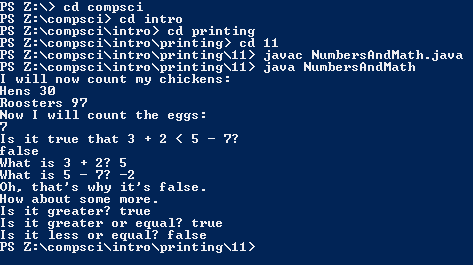# Assignment #11 and Numbers And Math

## Code

```    /// Name: Tim Chuang
/// Period: 7
/// Program Name: Numbers And Math
/// File Name: NumbersAndMath.java
/// Date Finished: 9/15/2015

class NumbersAndMath {

public static void main(String[]args) {

System.out.println("I will now count my chickens");
// + adds, / divides, - subtracts, * multiples, % takes the percentage
System.out.println( "Hens " + ( 25 + 30 / 6 ) );
System.out.println( "Roosters " + ( 100 - 25 * 3 % 4 ) );

System.out.println( "Now I will count the eggs:" );
// Same as above
System.out.println( 3 + 2 + 1 - 5 + 4 % 2 - 1 / 4 + 6 );

System.out.println( "Is it true that 3 + 2 < 5 - 7?" );
// Prints false because 5 > 2
System.out.println( 3 + 2 < 5 - 7 );
System.out.println( "What is 3 + 2? " + ( 3 + 2 ) );
System.out.println( "What is 5 - 7? " + ( 5 - 7 ) );
//
System.out.println( "Oh, that's why it's false." );
//
System.out.println( "How about some more." );
// Displays True or False depending on whether the value correct.
System.out.println( "Is it greater? " + ( 5 > -2 ) );
System.out.println( "Is it greater or equal? " + ( 5 >= -2 ) );
System.out.println( "Is it less or equal? " + ( 5 <= -2 ) );

}
}
```

### Picture of the output